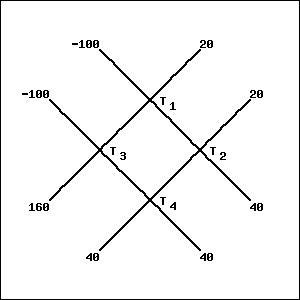In a grid of wires, the temperature at exterior mesh poins is maintained at constant values as shown in the figure. When the grid is in thermal equilibrium, the temperature at each interior mesh point is the average of the temperatures at the four adjacent points. For instance, Find the temperatures $T_1,$ $T_2,$ $T_3,$ $T_4,$ when the grid is in thermal equilibrium. $T_1=$ $T_2=$ $T_3=$ $T_4=$You can earn partial credit on this problem.